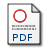# Field D* Pathfinding in Weighted Simplicial Complexes

Perkins, Simon James (2014) Field D* Pathfinding in Weighted Simplicial Complexes, PhD.PDF thesis.pdf Download (7MB)

## Abstract

The development of algorithms to efficiently determine an optimal path through a complex environment is a continuing area of research within Computer Science. When such environments can be represented as a graph, established graph search algorithms, such as Dijkstra’s shortest path and A*, can be used. However, many environments are constructed from a set of regions that do not conform to a discrete graph. The Weighted Region Problem was proposed to address the problem of finding the shortest path through a set of such regions, weighted with values representing the cost of traversing the region. Robust solutions to this problem are computationally expensive since finding shortest paths across a region requires expensive minimisation. Sampling approaches construct graphs by introducing extra points on region edges and connecting them with edges criss-crossing the region. Dijkstra or A* are then applied to compute shortest paths. The connectivity of these graphs is high and such techniques are thus not particularly well suited to environments where the weights and representation frequently change. The Field D* algorithm, by contrast, computes the shortest path across a grid of weighted square cells and has replanning capabilites that cater for environmental changes. However, representing an environment as a weighted grid (an image) is not space-efficient since high resolution is required to produce accurate paths through areas containing features sensitive to noise. In this work, we extend Field D* to weighted simplicial complexes – specifically – triangulations in 2D and tetrahedral meshes in 3D. Such representations offer benefits in terms of space over a weighted grid, since fewer triangles can represent polygonal objects with greater accuracy than a large number of grid cells. By exploiting these savings, we show that Triangulated Field D* can produce an equivalent path cost to grid-based Multi-resolution Field D*, using up to an order of magnitude fewer triangles over grid cells and visiting an order of magnitude fewer nodes. Finally, as a practical demonstration of the utility of our formulation, we show how Field D* can be used to approximate a distance field on the nodes of a simplicial complex, and how this distance field can be used to weight the simplicial complex to produce contour-following behaviour by shortest paths computed with Field D*.

Item Type: Electronic thesis or dissertation (PhD) pathfinding, Field D*, Weighted Region Problem Computing methodologies > Artificial intelligence 14 Feb 2014 10 Oct 2019 15:32 http://pubs.cs.uct.ac.za/id/eprint/924View Item